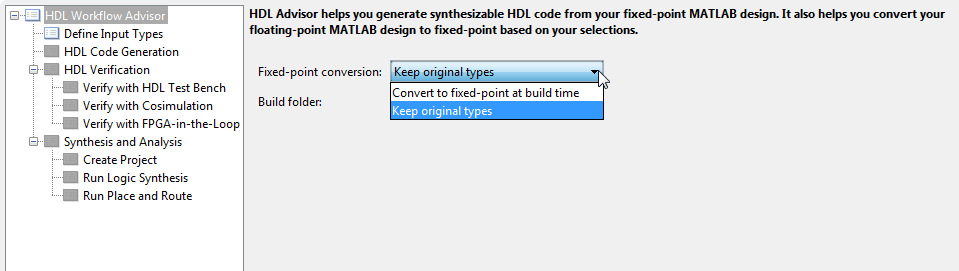# Working with Fixed-Point Code

This example shows HDL code generation from a fixed-point MATLAB® design that is ready for code generation.

### Introduction

The MATLAB code used in the example is an implementation of viterbi decoder modeled using fixed-point constructs.

```design_name = 'mlhdlc_viterbi'; testbench_name = 'mlhdlc_viterbi_tb'; ```
1. MATLAB Design: mlhdlc_viterbi

2. MATLAB testbench: mlhdlc_viterbi_tb

Open the design function mlhdlc_viterbi by clicking on the above link to notice the use of Fixed-Point Designer functions:

1. use of 'fi', 'numerictype', and 'fimath' for modeling fixed-point data types

2. use of 'bitget', 'bitsliceget', 'bitconcat' for modeling bit-wise operations

### Create a New Folder and Copy Relevant Files

Execute the following lines of code to copy the necessary example files into a temporary folder.

```mlhdlc_demo_dir = fullfile(matlabroot, 'toolbox', 'hdlcoder', 'hdlcoderdemos', 'matlabhdlcoderdemos'); mlhdlc_temp_dir = [tempdir 'mlhdlc_fixpt_design']; % create a temporary folder and copy the MATLAB files cd(tempdir); [~, ~, ~] = rmdir(mlhdlc_temp_dir, 's'); mkdir(mlhdlc_temp_dir); cd(mlhdlc_temp_dir); copyfile(fullfile(mlhdlc_demo_dir, [design_name,'.m*']), mlhdlc_temp_dir); copyfile(fullfile(mlhdlc_demo_dir, [testbench_name,'.m*']), mlhdlc_temp_dir); ```

### Create a New HDL Coder™ Project

```coder -hdlcoder -new fixpt_codegen ```

Next, add the file 'mlhdlc_viterbi.m' to the project as the MATLAB Function and 'mlhdlc_viterbi_tb.m' as the MATLAB Test Bench.

Refer to Get Started with MATLAB to HDL Workflow for a more complete tutorial on creating and populating MATLAB HDL Coder projects.

### Skip Fixed-Point Conversion

Launch the HDL Advisor and choose 'Keep original types' on the option 'Fixed-point conversion:'.The Floating-point to fixed-point conversion related step is removed from the workflow tree when we skip the conversion.

If your design is in floating-point, follow the instructions in Floating-Point to Fixed-Point Conversion and convert your design to fixed-point before moving onto the HDL code generation steps.

### Run HDL Code Generation

Right click on the 'Code Generation' step and choose the option 'Run this task' to run all code generation step directly.

Examine the generated HDL code by clicking on the hyperlinks in the Code Generation Log window.

### Try More Code Generation Options

As this is a large design with considerable number of functions you can try the option 'Generate instantiable code for functions' in the Advanced tab.

Re-examine the generated HDL code and compare it with the previous step.

### Clean up the Generated Files

You can run the following commands to clean up the temporary project folder.

```mlhdlc_demo_dir = fullfile(matlabroot, 'toolbox', 'hdlcoder', 'hdlcoderdemos', 'matlabhdlcoderdemos'); mlhdlc_temp_dir = [tempdir 'mlhdlc_fixpt_design']; clear mex; cd (mlhdlc_demo_dir); rmdir(mlhdlc_temp_dir, 's'); ```